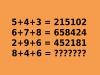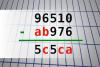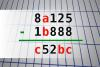BRAIN TEASERSBrain Teasers User Profile

# Roxana zavari

rank
20
points
2489
See full ranking list
short ranking list
 18 Betty Krpan 2831 19 Maryam Pouya 2506 20 Roxana zavari 2489 21 Jasmina Atarac 2417 22 Snezana Milanovic 2406
 Mathematical Puzzle: IF 5+4+... Mathematical Puzzle: IF 5+4+3=215102, 6+7+8=658424 and 2+9+6=452181 THEN 8+4+6=?Find number abc If 96510 - ab976 = 5c5ca find number abc. Multiple solutions may exist.Find number abc If 8a125 - 1b888 = c52bc find number abc. Multiple solutions may exist.